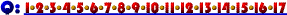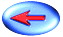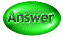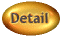17. Consider a manufacturing firm which uses natural gas to generate its own electricity. The electricity is used to run its manufacturing equipment. If the price of natural gas rises this would lead to:

1. a shift up in the marginal cost, average variable cost, and average fixed cost curves.
2. a shift up in the average fixed cost and average total cost curves.
3. a shift up in the marginal cost, average total cost, and average fixed cost curves.
4. a shift up in the marginal cost, average variable cost, and average total cost curves.
5. a shift up only in the average total cost curve.Click Here if you don't see topics listed to the left and below.